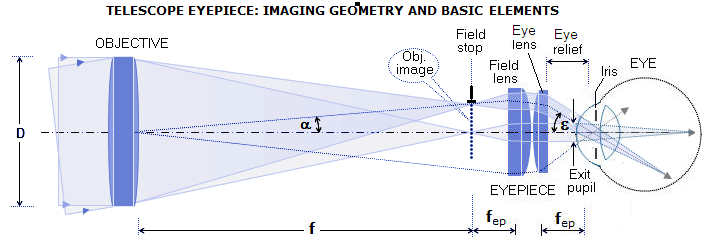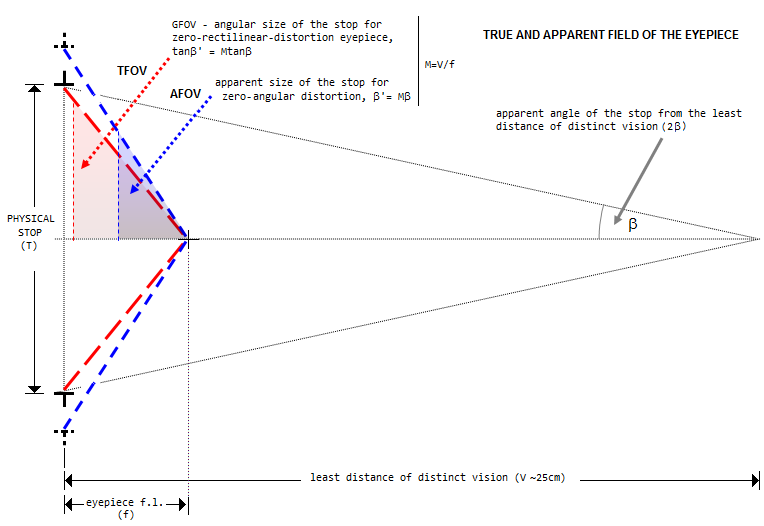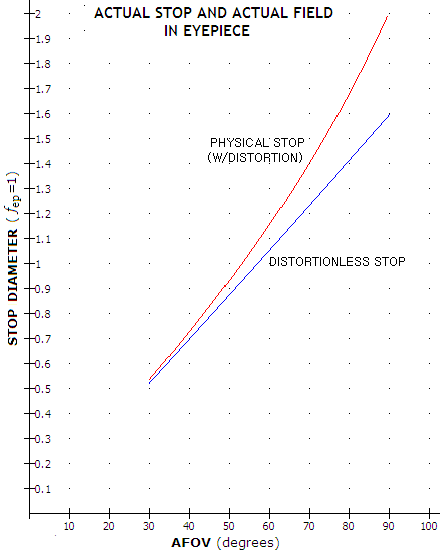telescopeѲptics.net          ▪▪▪▪                                             CONTENTS

11. MISCELLANEOUS OPTICS

# 12. TELESCOPE EYEPIECE

## 12.1. Eyepiece functions

The image formed by the telescope objective is real, and can be observed directly. However, as explained in
1.4. Main functions of a telescope, its magnification is limited to ~ƒ/250, ƒ being the objective focal length. Also, since an average eye can't focus properly looking at objects closer than ~25cm, it can grasp only a fraction of light coming from the image formed by the objective (FIG. 6). It is telescope eyepiece that solves both of these problems. It increases telescope magnification by a 250/ƒ
e factor (ƒe being the eyepiece f.l. in mm), and brings all the light emitted by the object-image to the eye pupil (FIG. 7).

Telescope eyepiece is a complex positive lens system placed between the eye and the image formed by the objective. If the object image formed by the objective is located at the ocular's front focal plane, the eyepiece images every image point at infinity - in other words, it transforms (desirably) spherical wavefronts emerging from the object image's points into flat wavefronts merging at the location of the eyepiece exit pupil (in terms of rays, the eyepiece turns diverging cones emerging from the objective's image into collimated pencils of light, FIG. 209). These wavefronts enter the eye, which transforms them into near-spherical and have them focus onto the retina, creating the final magnified apparent image.

The figure illustrates the basic properties of a telescope eyepiece: focal length, field of view (directly determined by its field stop size), exit pupil and eye reliefFIGURE
209
: (A) Standard eyepiece with the object-image formed by the objective at its front focal plane turns the diverging cone from every point source of the object-image into a collimated pencil. Image of the objective formed by the eyepiece (dotted lines) is the exit pupil, where the pencils of collimated light merge, making possible for the eye - with the eye's iris placed approximately at the exit pupil - to receive all the light emitted by the object image. Since the pencils entering the eye are collimated, the image formed by the eye is projected at infinity. With the objective image magnification given by the image distance ƒƒe/(ƒ-ƒe) to the object distance ƒ+ƒe ratio, the exit pupil diameter is Pex=Dƒƒe/(ƒ2-ƒe2) or, practically, Pex=Dƒe/ƒ=ƒe/F~D/M, M being the telescope magnification, and F the telescope F#. Telescope magnification M=tanε/tanα, ε being the apparent eyepiece image angle, and α the apparent object angle. The distance ER between the eye lens and the exit pupil is called eye relief. For full field edge illumination, the front lens needs to be somewhat larger than object-image in the focal plane (specific value varies with the eyepiece type - i.e. stop to field lens separation - and telescope ƒ-ratio).(B) Smyth-type eyepiece has a negative front lens - in effect an integrated Barlow lens - separated from the positive lens froup following it. Smyth lens forms a new focus, generally farther away from the objective, also magnifying the image. In order to produce magnification corresponding to the eyepiece designated focal length, the positive lens group has to have focal length larger by the factor of Smyth lens magnification. Main benefit of this configuration - whose origins date back to the 19. century - is the reduction of off axias astigmatism by offsetting the "regular" astigmatism of the positive-power lens group by that of the Smyth lens. Consequently, it has become configuration of choice for the widefield and ultrawidefield eyepieces.
Basic properties of the configuration are: (1) Smyth lens acts a focal tele-extender, magnifying objective's image, (2) in order to produce the designated eyepiece focal length, the positive lens group has to have the actual focal length equal to the designated eyepiece focal length multiplied with the Smyth lens magnification factor, (3) the field stop diameter for the designated eyepiece focal length is determined by the actual field stop, in front of the positive lens group, and equals the actual stop divided by the Smyth lens magnification factor (so, when, for instance, Tele Vue lists the field stop of its "Ethos" 21mm unit as 36.2mm, it is the virtual field stop at the original focus of the objective, obtained by dividing the actual, internal field stop by the Smyth lens' - in this case, the Smyth lens group, that inludes a positive meniscus - magnification).

1. FOCAL LENGTH

The main eyepiece parameter, determining its basic function - magnification - is its focal length ƒe. Normally, it cannot be directly measured as the separation between the lens and image of a distant object, because the lens (i.e. eyepiece) thickness is large compared to the f.l., and the effective entrance pupil is projected into the eyepiece. From Pex=ƒe/F it follows that ƒe=FPex, i.e. eyepiece focal length is given by a product of the telescope focal ratio and exit pupil diameter. That, of course, requires knowledge of telescope's focal length a precise measurement of the eyepiece exit pupil.

An alternative method of establishing ƒe is measuring the image size of a relatively distant object vs. size of its image produced by the eyepiece. From the geometry of image formation (FIG. 7), the proportion between object and image height ho and hi, respectively, equals that between object and image lens separation, So and Si, in the same order, thus

Si = Sohi/ho,

irrespectively of lens thickness. With focal length defined by Eq. 1.4 as ƒ=SiSo/(Si+So), and hi/ho=Si/So, the relation between image separation and focal length is Si/ƒ=(Si+So)/So=(Si/So)+1=1+(hi/ho) and

ƒ = Si/[1+(hi/ho)] = So/[1+(ho/hi)]

Thus eyepiece focal length can be obtained from the measurements of the object size (ho), image size (hi) and object distance (So). The object's image can be measured either as projected on a piece of paper, or directly as seen at the eye lens. Best object type is a bright, uniform surface with well defined boundaries, such as a well lit window. Size of the image should be kept at the minimum allowing accurate measurement, in order to minimize distortion (which tends to enlarge outer field portion, thus to result in shorter than actual eyepiece focal length if that portion of the field is used). Also, greater object distance - 50 to 100 focal lengths - will minimize the error of (most likely) adding to the object distance, measured from the field lens, a small differential between that point and second principal plane of the eyepiece.

2. FIELD OF VIEW

The next important eyepiece parameter is its field of view. Limit to the eyepiece apparent field is set by its field stop, an axially centered opening in front of the field lens, which for focused eyepiece coincides with the objective's image plane. Angular size of the field stop as seen from the center of the entrance pupil (α, FIG. 209) is called true field of view (TFOV), and its angular size as seen through the eyepiece (ε, FIG. 209) is apparent field of view (AFOV) of a telescope (here, both are presented as field radius; often times, the terms are also used for the field diameter).Illustration above shows the geometry of eyepiece's apparent and true field of view. Since eyepiece effectively allows the eye to observe objective's image from the distance equaling eyepiece's focal length, the lenses can be entirely left out. Eyepiece magnification M is given by a factor of reduction of observing distance vs. naked eye, equaling V/f (which multiplied by magnification of the objective, f'/V, f' being the objective's f.l., gives telescope magnification). With zero rectilinear distortion, condition of which is that any zonal height "h" in the field stop becomes M times larger through the eyepiece, i.e. that the tangent of its magnified angle is M times larger than tangent of the unmagnified angle. The corresponding angular size of the field stop in the eyepiece is the geometric field of view (GFOV). Deviation from this condition causes curving of straight lines in the eyepiece image. With zero angular distortion, every zonal angle in the stop is M times larger in the eyepiece image and, since multiplied angle is larger than the angle corresponding to its multiplied tangent, the apparent stop size with zero angular distortion is always larger than with zero rectilinear distortion, i.e. requires non-zero (positive) rectilinear distortion. Deviation from zero angular distortion causes elongation of shapes, either vertical (positive), or horizontal (negative). Actual apparent stop size can be anywhere from smaller than GFOV (w/negative rectilinear and angular distortion) to larger than zero-angular-distortion angular size (w/positive rectilinear and angular distortion), but usually is close to the zero-angular-distortion size.

The AFOV/TFOV ratio approximates telescope magnification; however, its approximate accuracy varies with the degree of eyepiece image distortion. Since magnification is defined as apparent image size vs. apparent object size, it is given by the ratio of tangents, or M=tan(AFOV/2)/tan(TFOV/2). With the TFOV being always a small angle, image distortion is negligible, and the actual image angle is practically given by tan(TFOV/2)=T/2ƒ, T being the diameter of eyepiece field stop (being a small angle, it is also closely approximated in degrees by TFOV~180T/2ƒπ).However, the eyepiece field of view, much larger angularly, suffers from significant distortion. In the telescope eyepiece, it is usually positive distortion, which means that image magnification increases exponentially with the image point height. In effect, the outer image portion is stretched out and seen at a magnification higher than that for the inner image portions (this may and may not be accompanied with spherical aberration of exit pupil). In effect, AFOV inflated by distortion implies the field stop - and true field - larger by the factor of distortion than what it actually is. That is relatively insignificant with small AFOV eyepieces (~5% average in a conventional ~45° eyepiece), but since distortion increases with the third power of the angle, it can be a factor in the wide-field varieties. For instance, a zero-distortion 10mm 60° AFOV eyepiece would have 11.5mm field stop diameter, while one with 10% distortion would have it ~12.6mm.

The field stop diameter corresponding to the actual (distortionless) angular field is always smaller than the actual stop. With the usual magnitude of distortion ranging from up to 5% with 40° FOV to up to 20% with 80°, it is approximated by T~ƒeAFOV/[58-(AFOV/58)], for AFOV in degrees (plot at left).

Details seen in the eyepiece as extended are larger than 3 arc minutes. For the average eye, smaller details don't have recognizable shape, even when they don't appear point-like. Airy disc diameter in the eyepiece is 4.6F/ƒe arc minutes, for 550nm wavelength, with F being the telescope focal ratio. This sets the minimum eyepiece focal length needed to begin recognizing it as a spot at ƒe~1.5F (assuming sufficiently bright star). This, of course, can and does vary individually. In field conditions, the minimum angular size needed by the eye for shape recognition is closer to 5 arc minutes, putting the corresponding eyepiece f.l. at about ƒe~F.

3. EXIT PUPIL SIZE

Another important eyepiece-related parameter is the size of its exit pupil. It directly determines image brightness level relative to the object, as well as the level of eye aberrations. The size of eyepiece exit pupil is inversely proportional to telescope magnification. For the relative magnification m, in units of aperture diameter, eyepiece exit pupil is given by 1/m for aperture in mm, and by 25.4/m for aperture in inches. So, for instance, relative magnification of 0.5 per millimeter of aperture (50x for D=100mm, with m=0.5), results in 2mm exit pupil diameter, and so does 12.7x per inch of aperture magnification (m=12.7).

In terms of the telescope focal ratio F=ƒobj/D, exit pupill diameter is given by P=ƒe/F, where ƒe is the eyepiece focal length. Another important number related to the exit pupil diameter is the Airy disc angular diameter, given by 4.6/P(mm) in arc minutes.

At the exit pupil size larger than about 2mm in diameter, eye aberrations begin to dominate diffraction effect, increasing progressively with the pupil size. Thus, telescopic resolution is aberrations-limited for exits pupils larger than ~2mm, and diffraction-limited for smaller pupils.

4. EYE RELIEF

Eyepiece eye relief - the separation between the eye and exit pupil - is mainly important for observing convenience. As with most anything else, too little is as undesirable as too much. Short eye relief, typical of the short f.l. conventional eyepieces, prevents observer from placing eye at the exit pupil, thus effectively reducing apparent field. It also causes eye strain, detrimental to the quality of observing. Too long eye relief, often encountered with long f.l. eyepieces, especially when used with a conventional (not telecentric) Barlow lens, makes it difficult to find exit pupil and keep eye pupil on it. Size of eye relief varies mainly with the eyepiece type and design (FIG. 215 and FIG. 213), although it may vary somewhat within them as well.

11. Solar telescope